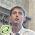## Sunday, October 24, 2010

### Planning problems in the cloud

Everybody is talking about the cloud these days, but it too suffers from planning problems.
So I created a new example this weekend for Drools Planner to deal with those planning problems: CloudBalance (available on trunk).

The problem is simple: there are a number of computers and a number of processes, which we want to run on the computers. Every computer should be able to handle the sum of the minimal hardware requirements of all its processes.

Here's part of an example with 100 computers and 200 processes. If I only use a deterministic algorithm, which plans the biggest processes first, I get this unfeasible planning after 1.6 seconds:As you can see, some processes don't meet their minimal CPU requirements.
On the same example, if I continue planning with tabu search on that original solution, I get this feasible planning after 3.4 seconds:All processes meet their minimal hardware requirements. And if we give it more time, it will try to shut down computers and save power costs, while still meeting the minimal hardware requirements of all processes.#### 4 comments:

1.And this is the IP formulation for solving this assignment problem. (Note that a column oriented approach might be more interesting/efficient!)

let i = 1 .. n be the index for the processes
let j = 1 .. m be the index for the machines

let C(i) be the required CPU speed for the process and C(j) be the available CPU speed for the machines

Similarly, R(i) and R(j) indicate required/available ram, N(i) and N(j) indicate required/available network

define the binary decision variables x_ij and y_j.

Let x_ij = 1 if process i is assigned to machine j, and x_ij = 0 otherwise

Let y_j = 1 if machine j is used, y_j = 0 otherwise

Minimize Sum_j y_j

Subject to:

Sum_j x_ij = 1 forall i (all processes should be assigned exactly once)

Sum_i C(i)*x_ij <= n*C(j)*y_j forall j (sum of processes on a machine should not exceed CPU speed)

Sum_i R(i)*x_ij <= n*R(j)*y_j forall j

Sum_i N(i)*x_ij <= n*N(j)*y_j forall j

x_ij = binary forall i,j and y_j binary forall j

You could feed this IP program to any IP solver (cplex, gurobi, ...)

2.Whoops, you should drop the 'n' in the capacity constraints, i.e. :

Sum_i C(i)*x_ij <= C(j)*y_j forall j
Sum_i R(i)*x_ij <= R(j)*y_j forall j
Sum_i N(i)*x_ij <= N(j)*y_j forall j

3.Such IP maths are pretty hard to read for a plain old java object oriented programmer like me... but if I am not mistaken, the IP solver listing doesn't include the "cost" soft constraint.

In my experience, IP solvers don't scale up. Have you tried implementing the ITC2007 examination track in cplex or gurobi? I believe several did, but none made the finalists (and not in the other tracks either IIRC).

BTW, is it possible to reuse a peice of javacode in such IP solver maths? For example:
public boolean isHoliday(Date date, Country country);

4.